Courses

# Tree (Basic Level) - 1

## 10 Questions MCQ Test Question Bank for GATE Computer Science Engineering | Tree (Basic Level) - 1

Description
This mock test of Tree (Basic Level) - 1 for Computer Science Engineering (CSE) helps you for every Computer Science Engineering (CSE) entrance exam. This contains 10 Multiple Choice Questions for Computer Science Engineering (CSE) Tree (Basic Level) - 1 (mcq) to study with solutions a complete question bank. The solved questions answers in this Tree (Basic Level) - 1 quiz give you a good mix of easy questions and tough questions. Computer Science Engineering (CSE) students definitely take this Tree (Basic Level) - 1 exercise for a better result in the exam. You can find other Tree (Basic Level) - 1 extra questions, long questions & short questions for Computer Science Engineering (CSE) on EduRev as well by searching above.
QUESTION: 1

### A binary tree T has n leaf nodes. The number of nodes of degree 2 in T is

Solution:

Because for 2 degree node, every time ‘2’ leafs are added and number of nodes increases is '1'. So number of nodes with degree 2 is always one less than number f leafs present in tree.

QUESTION: 2

### Which of the following sequences denotes the post order traversal sequence of the tree of above question?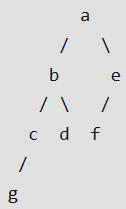Solution: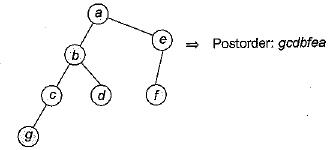QUESTION: 3

### In the balanced binary tree in the figure given below, how many nodes will become unbalanced when a node is inserted as a child of the node "g”?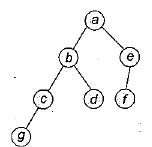Solution: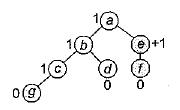When a node is added as a child of g.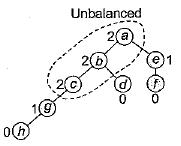Nodes a, b and c become unbalanced.

QUESTION: 4

A binary search tree is generated by inserting in order of the following integers: 50, 15, 62, 5, 20, 58, 91, 3, 8, 37, 60, 24. The number of nodes in the left subtree and right subtree of the root respectively is

Solution:

The binary search tree is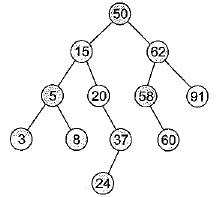The number of nodes in the left subtree and right subtree of the root respectively is (7, 4).

QUESTION: 5

A binary search tree contains the values 1,2,3, 4, 5, 6, 7, 8. The tree is traversed in pre-order and the values are printed out. Which of the following sequences is a valid output?

Solution:

(a) 53124786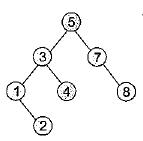6 can not be child of 8.
(b) 5312648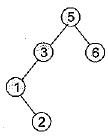4 can not be child of 6.
(c) 53241678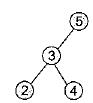1 can not be child of 4 or 5.

QUESTION: 6

Which of the following statements is false?

Solution:

A labelled rooted binary tree can not be constructed uniquely when inorder traversal is given along with post-order or pre-order traversal.

QUESTION: 7

A complete n-ary tree is one in which every node has 0 or n sons. If x is the number of internal nodes of a complete n-ary tree, the number of leaves in it is given by

Solution:

In n-ary tree the total number of vertices are m = nx + 1
Where x: number of internal nodes and let l be the number of leaf nodes.
x + l = nx +1
l = (n - 1)x + 1
So number of leaf nodes in n-ary tree with x internal nodes is (n - 1) x + 1.

QUESTION: 8

Consider the following nested representation of binary trees: (X Y Z) indicates Y and Z are the left and right subtrees, respectively, of node X. Note that Y and Z may be NULL, or further nested. Which of the following represents a valid binary tree?

Solution:

(1 (2 3 4) (5 6 7))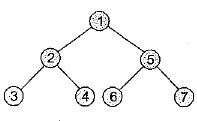QUESTION: 9

Let LASTPOST, LASTIN and LASTPRE denote the last vertex visited in a postorder, inorder and preorder traversal. Respectively, of a complete binary tree. Which of the following is always true?

Solution:

Because of complete binary tree option (b) is always correct.

QUESTION: 10

The number of leaf nodes in a rooted tree of n nodes, with each node having 0 or 3 children is

Solution:

In m-ary tree
Total number of nodes = m(internal nodes) + 1
Here, n = 3i + 1
Total nodes = Internal nodes + Leaf nodes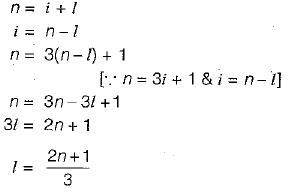Where l is leaf nodes.